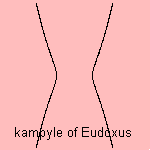# kampyle of Eudoxus

## quarticThis quartic curve has been studied by Eudoxus of Cnide (406-355 BC), an astronomer, philosopher and mathematician who was a pupil of Plato.

Eudoxus
worked on the curve in relation to the classical problem of the duplication of the cube.
Let there be a circle C through O with radius √1/2, which cuts the kampyle in P.
Then OP has as length 3√2In polar coordinates the curve can be written as r = cos -2 φ.

The kampyle 1) is also named Clairaut's curve.

And the kampyle is:

Its Cartesian equation resembles the equation of the lemniscate of Gerono.

notes

1) Kampyle (Gr.) = curved